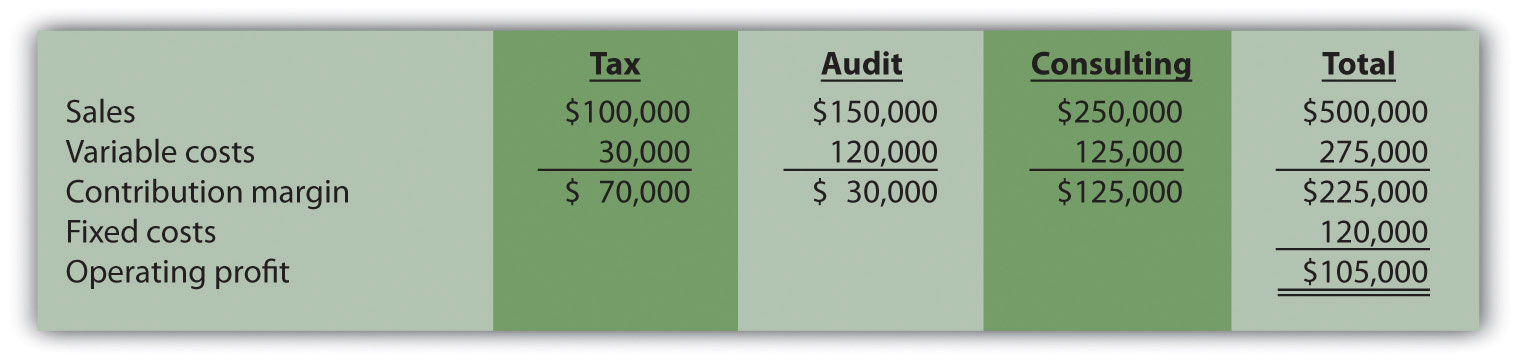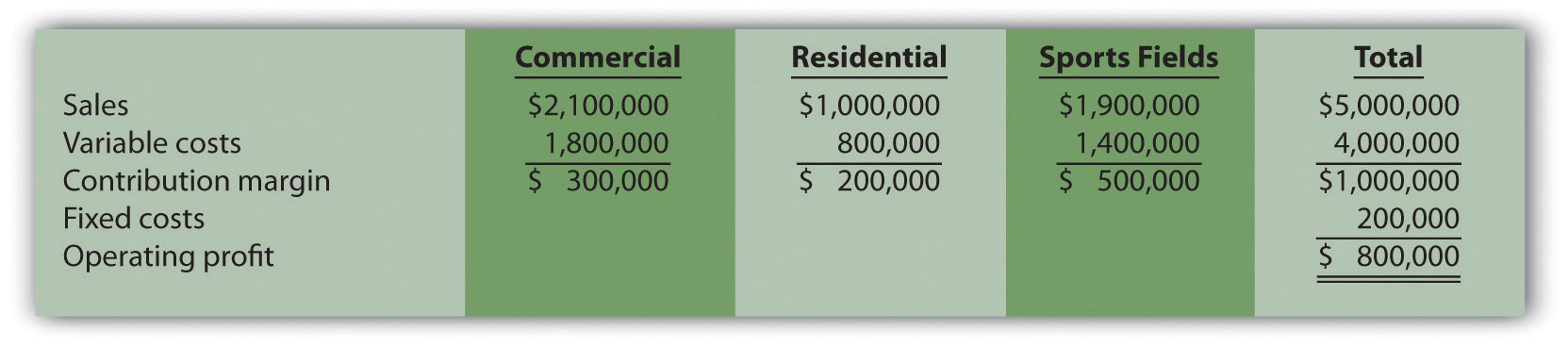## 6.2 Cost-Volume-Profit Analysis for Multiple-Product and Service Companies

### Learning Objective

1. Perform cost-volume-profit analysis for multiple-product and service companies.

Question: Although the previous section illustrated cost-volume-profit (CVP) analysis for companies with a single product easily measured in units, most companies have more than one product or perhaps offer services not easily measured in units. Suppose you are the manager of a company called Kayaks-For-Fun that produces two kayak models, River and Sea. What information is needed to calculate the break-even point for this company?

Answer: The following information is required to find the break-even point:

### Review Problem 6.2

International Printer Machines (IPM) builds three computer printer models: Inkjet, Laser, and Color Laser. Information for these three products is as follows:

 Inkjet Laser Color Laser Total Selling price per unit $250$400 $1,600 Variable cost per unit$100 $150$   800 Expected unit sales (annual) 12,000 6,000 2,000 20,000 Sales mix 60 percent 30 percent 10 percent 100 percent

Total annual fixed costs are $5,000,000. Assume the sales mix remains the same at all levels of sales. 1. 1. How many printers in total must be sold to break even? 2. How many units of each printer must be sold to break even? 2. 1. How many printers in total must be sold to earn an annual profit of$1,000,000?
2. How many units of each printer must be sold to earn an annual profit of $1,000,000? Solution to Review Problem 6.2 Note: All solutions are rounded. 1. 1. IPM must sell 20,408 printers to break even: 2. As calculated previously, 20,408 printers must be sold to break even. Using the sales mix provided, the following number of units of each printer must be sold to break even: 2. 1. IPM must sell 24,490 printers to earn$1,000,000 in profit:

2. As calculated previously, 24,490 printers must be sold to earn $1,000,000 in profit. Using the sales mix provided, the following number of units for each printer must be sold to earn$1,000,000 in profit:

## Finding the Break-Even Point and Target Profit in Sales Dollars for Multiple-Product and Service Companies

A restaurant like Applebee’s, which serves chicken, steak, seafood, appetizers, and beverages, would find it difficult to measure a “unit” of product. Such companies need a different approach to finding the break-even point. Figure 6.4 "Type of Good or Service Determines Whether to Calculate Break-Even Point and Target Profit Points in Units or Sales Dollars" illustrates this point by contrasting a company that has similar products easily measured in units (kayaks) with a company that has unique products (meals at a restaurant) not easily measured in units.

## Break-Even Point in Sales Dollars and the Weighted Average Contribution Margin Ratio

Question: For companies that have unique products not easily measured in units, how do we find the break-even point?

Answer: Rather than measuring the break-even point in units, a more practical approach for these types of companies is to find the break-even point in sales dollars. We can use the formula that follows to find the break-even point in sales dollars for organizations with multiple products or services. Note that this formula is similar to the one used to find the break-even point in sales dollars for an organization with one product, except that the contribution margin ratio now becomes the weighted average contribution margin ratio.

### Key Equation

For example, suppose Amy’s Accounting Service has three departments—tax, audit, and consulting—that provide services to the company’s clients. Figure 6.5 "Income Statement for Amy’s Accounting Service" shows the company’s income statement for the year. Amy, the owner, would like to know what sales are required to break even. Note that fixed costs are known in total, but Amy does not allocate fixed costs to each department.

Figure 6.5 Income Statement for Amy’s Accounting ServiceThe contribution margin ratio differs for each department:

 Tax 70 percent (= $70,000 ÷$100,000) Audit 20 percent (= $30,000 ÷$150,000) Consulting 50 percent (= $125,000 ÷$250,000)

Question: We have the contribution margin ratio for each department, but we need it for the company as a whole. How do we find the contribution margin ratio for all of the departments in the company combined?

Answer: The contribution margin ratio for the company as a whole is the weighted average contribution margin ratioThe total contribution margin divided by total sales.. We calculate it by dividing the total contribution margin by total sales. For Amy’s Accounting Service, the weighted average contribution margin ratio is 45 percent (= $225,000 ÷$500,000). For every dollar increase in sales, the company will generate an additional 45 cents ($0.45) in profit. This assumes that the sales mix remains the same at all levels of sales. (The sales mix here is measured in sales dollars for each department as a proportion of total sales dollars.) Now that you know the weighted average contribution margin ratio for Amy’s Accounting Service, it is possible to calculate the break-even point in sales dollars: Amy’s Accounting Service must achieve$266,667 in sales to break even.The weighted average contribution margin ratio can also be found by multiplying each department’s contribution margin ratio by its proportion of total sales. The resulting weighted average contribution margin ratios for all departments are then added. The calculation for Amy’s Accounting Service is as follows:45 percent weighted average contribution margin ratio = (tax has 20 percent of total sales × 70 percent contribution margin ratio) + (audit has 30 percent of total sales × 20 percent contribution margin ratio) + (consulting has 50 percent of total sales × 50 percent contribution margin ratio)Thus 45 percent = 14 percent + 6 percent + 25 percent.

## Target Profit in Sales Dollars

Question: How do we find the target profit in sales dollars for companies with products not easily measured in units?

Answer: Finding the target profit in sales dollars for a company with multiple products or services is similar to finding the break-even point in sales dollars except that profit is no longer set to zero. Instead, profit is set to the target profit the company would like to achieve.

### Key Equation

For example, assume Amy’s Accounting Service would like to know sales dollars required to make $250,000 in annual profit. Simply set the target profit to$250,000 and run the calculation:

Amy’s Accounting Service must achieve $822,222 in sales to earn$250,000 in profit.

## Important Assumptions

Question: Several assumptions are required to perform break-even and target profit calculations for companies with multiple products or services. What are these important assumptions?

Answer: These assumptions are as follows:

• Costs can be separated into fixed and variable components.
• Contribution margin ratio remains constant for each product, segment, or department.
• Sales mix remains constant with changes in total sales.

These assumptions simplify the CVP model and enable accountants to perform CVP analysis quickly and easily. However, these assumptions may not be realistic, particularly if significant changes are made to the organization’s operations. When performing CVP analysis, it is important to consider the accuracy of these simplifying assumptions. It is always possible to design a more accurate and complex CVP model. But the benefits of obtaining more accurate data from a complex CVP model must outweigh the costs of developing such a model.

## Margin of Safety

Question: Managers often like to know how close expected sales are to the break-even point. As defined earlier, the excess of projected sales over the break-even point is called the margin of safety. How is the margin of safety calculated for multiple-product and service organizations?

Answer: Let’s return to Amy’s Accounting Service and assume that Amy expects annual sales of $822,222, which results in expected profit of$250,000. Given a break-even point of $266,667, the margin of safety in sales dollars is calculated as follows: Thus sales revenue can drop by$555,555 per year before the company begins to incur a loss.

### Key Takeaways

• The key formula used to calculate the break-even or target profit point in units for a company with multiple products is as follows. Simply set the target profit to $0 for break-even calculations, or to the appropriate profit dollar amount for target profit calculations. • The formula used to find the break-even point or target profit in sales dollars for companies with multiple products or service is as follows. Simply set the “Target Profit” to$0 for break-even calculations, or to the appropriate profit dollar amount for target profit calculations:

### Review Problem 6.3

Ott Landscape Incorporated provides landscape maintenance services for three types of clients: commercial, residential, and sports fields. Financial projections for this coming year for the three segments are as follows:Assume the sales mix remains the same at all levels of sales.

1. How much must Ott Landscape have in total sales dollars to break even?
2. How much must Ott Landscape have in total sales dollars to earn an annual profit of $1,500,000? 3. What is the margin of safety, assuming projected sales are$5,000,000 as shown previously?

Solution to Review Problem 6.3

1. Sales of $1,000,000 are required to break even: *Weighted average contribution margin ratio =$1,000,000 ÷ $5,000,000 = 20 percent or 0.20. 2. Sales of$8,500,000 are required to make a profit of $1,500,000: 3. The margin of safety is$4,000,000 in sales: